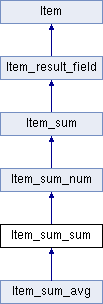My Project
Item_sum_sum Class Reference
Inheritance diagram for Item_sum_sum:List of all members.

## Public Member Functions

Item_sum_sum (Item *item_par, bool distinct)
Item_sum_sum (THD *thd, Item_sum_sum *item)
enum Sumfunctype sum_func () const
void clear ()
double val_real ()
longlong val_int ()
Stringval_str (String *str)
my_decimalval_decimal (my_decimal *)
enum Item_result result_type () const
void reset_field ()
void update_field ()
void no_rows_in_result ()
const char * func_name () const
Itemcopy_or_same (THD *thd)

## Protected Member Functions

void fix_length_and_dec ()

## Protected Attributes

Item_result hybrid_type
double sum
my_decimal dec_buffs 
uint curr_dec_buff

## Constructor & Destructor Documentation

 Item_sum_sum::Item_sum_sum ( THD * thd, Item_sum_sum * item )
Todo:
check if the following assignments are really needed

## Member Function Documentation

 void Item_sum_sum::no_rows_in_result ( ) ` [inline, virtual]`

Mark an aggregate as having no rows.

This function is called by the execution engine to assign 'NO ROWS FOUND' value to an aggregate item, when the underlying result set has no rows. Such value, in a general case, may be different from the default value of the item after 'clear()': e.g. a numeric item may be initialized to 0 by clear() and to NULL by no_rows_in_result().

Reimplemented from Item_sum.

Reimplemented in Item_sum_avg.

 void Item_sum_sum::update_field ( ) ` [virtual]`

calc next value and merge it with field_value.

Implements Item_sum.

Reimplemented in Item_sum_avg.

The documentation for this class was generated from the following files: